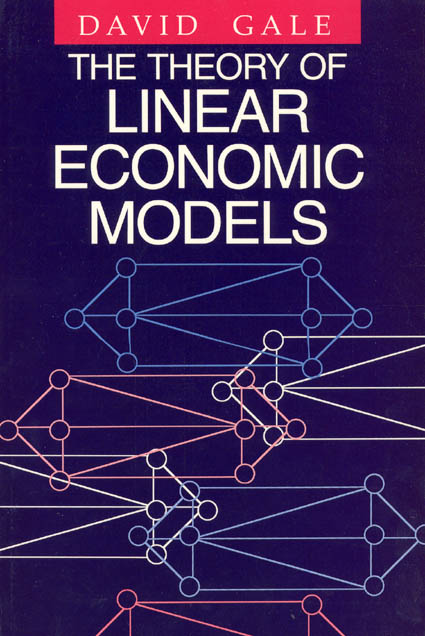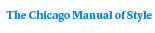# The Theory of Linear Economic Models

David Gale352 pages | 6 x 9 | © 1960
Paper \$34.00 ISBN: 9780226278841 Published February 1989
In the past few decades, methods of linear algebra have become central to economic analysis, replacing older tools such as the calculus. David Gale has provided the first complete and lucid treatment of important topics in mathematical economics which can be analyzed by linear models. This self-contained work requires few mathematical prerequisites and provides all necessary groundwork in the first few chapters. After introducing basic geometric concepts of vectors and vector spaces, Gale proceeds to give the main theorems on linear inequalities—theorems underpinning the theory of games, linear programming, and the Neumann model of growth. He then explores such subjects as linear programming; the theory of two-person games; static and dynamic theories of linear exchange models, including problems of equilibrium prices and dynamic stability; and methods of play, optimal strategies, and solutions of matrix games. This book should prove an invaluable reference source and text for mathematicians, engineers, economists, and those in many related areas.
Table of Contents• Contents
Contents
Preface
List of Notations
Chapter 1. Linear Programming: Examples, Definitions, and Statements of the Principal Theorems
1. Examples
The diet problem
The transportation problem
Production to meet given demand at minimum cost
Production to maximize income from given resources
2. Duality and prices
3. Further interpretation of duality
4. Price equilibrium
Bibliographical notes
Exercises
Chapter 2. Real Linear Algebra
1. Vector
2. Scalar product, matrices, linear equations
3. Real linear equations and inequalities
4. Basic solutions of equations
5. Geometry of linear inequalities. Convex cones
6. Extreme vectors and extreme solutions
7. Convex sets and polytopes
Bibliographical notes
Exercises
Chapter 3. The Theory of Linear Programming
1. Definitions
2. The duality theorems
3. The equilibrium theorems
4. Basic solutions
5. An application: allocation of resources in a competitive economy
Bibliographical notes
Exercises
Chapter 4. Computation. The Simplex Method
1. Solving simultaneous equations and inverting a matrix
2. The simplex method for linear programming. Discussion
3. Theory of the simplex method
4. Some numerical examples
5. Nonnegative solutions of linear equations
6. Solving linear inequalities
7. Degeneracy. The generalized simplex method
Bibliographical notes
Exercises
Chapter 5. Integral Linear Programming
1. Examples
Transportation problem with indivisible commodity
The optimal-assignment problem
2. Flows in networks
3. The simple-assignment problem
4. The transshipment problem
5. The optimal-assignment problem
6. A problem related to optimal assignment. Price equilibrium
7. The transportation problem
8. Other examples: shortest route; the caterer
9. Concluding remarks and open questions
Bibliographical notes
Exercises
Chapter 6. Two-person Games: Examples, Definitions, and Elementary Theory
1. First examples and definitions
Odds and evens (matching pennies)
Morra
2. Further examples of matrix games
Goofspiel
Bluffing
A, B, C
3. Solutions of games. Mixed strategies
4. Value of a game and optimal strategies
5. Some infinite games
Continuous bluffing
Duels
The oil prospector (a game against nature)
The bomber and the submarine
High number
Low number
7. Symmetric games
8. Proof of the fundamental theorem
Appendix to Chapter 6: A geometric "proof" of the fundamental theorem of game theory
Bibliographical notes
Exercises
Chapter 7. Solutions of Matrix Games
1. Relation between matrix games and linear programming
2. Solving games by the simplex method
3. Optimal strategies
4. Solutions
5. Examples
6. The structure of symmetric games
7. Constructing a game with prescribed solutions
8. Basic optimal strategies
9. A method of "learning" a game
10. Convergence of the learning method
Bibliographical notes
Exercises
Chapter 8. Linear Models of Exchange
1. Examples
The simple exchange model. The price problem
The simple linear model of international trade
2. Equilibrium for the exchange model
3. Dynamic theory
4. Dynamics in the reducible case
5. Price equilibrium for linear exchange models
6. An example of price equilibrium
7. Uniqueness of equilibrium prices
Bibliographical notes
Exercises
Chapter 9. Linear Models of Production
1. The simple linear production model
2. A dynamic property of the simple model
3. The Leontief model
4. The general linear production model. Efficient points
5. Von Neumann’s expanding model
6. Some examples
7. The expanding simple model
Bibliographical notes
Exercises
Bibliography
Index